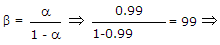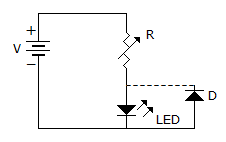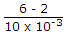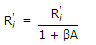Courses

# Test: Electronic Devices And Circuits - 1

## 25 Questions MCQ Test Electronic Devices | Test: Electronic Devices And Circuits - 1

Description
This mock test of Test: Electronic Devices And Circuits - 1 for Electrical Engineering (EE) helps you for every Electrical Engineering (EE) entrance exam. This contains 25 Multiple Choice Questions for Electrical Engineering (EE) Test: Electronic Devices And Circuits - 1 (mcq) to study with solutions a complete question bank. The solved questions answers in this Test: Electronic Devices And Circuits - 1 quiz give you a good mix of easy questions and tough questions. Electrical Engineering (EE) students definitely take this Test: Electronic Devices And Circuits - 1 exercise for a better result in the exam. You can find other Test: Electronic Devices And Circuits - 1 extra questions, long questions & short questions for Electrical Engineering (EE) on EduRev as well by searching above.
QUESTION: 1

### At room temperature the current in an intrinsic semiconductor is due to

Solution:

Intrinsic material has equal number of holes and electrons.

QUESTION: 2

### Work function is the maximum energy required by the fastest electron at 0 K to escape from the metal surface.

Solution:

Work function is the minimum energy required by the fastest electron at 0 K to escape from the metal surface.

QUESTION: 3

### The most commonly used semiconductor material is

Solution:

Germanium is rarely used.

QUESTION: 4

In which of these is reverse recovery time nearly zero?

Solution:

In schottky diode there is no charge storage and hence almost zero reverse recovery time.

QUESTION: 5

A transistor has a current gain of 0.99 in the CB mode. Its current gain in the CC mode is

Solution:Current gain = 1 + β = 100.

QUESTION: 6

In p-n-p transistor the current IE has two components viz. IEP due to injection of holes from p-region to n-region and IE due to injection of electrons from n-region to p-region. Then

Solution:

Emitter is p-type in p-n-p transistor.

Therefore holes are majority carriers.

QUESTION: 7

In an n channel JFET, the gate is

Solution:

Since channel is n type gate must be p type.

QUESTION: 8

The amount of photoelectric emission current depends on

Solution:

Only the intensity of incident radiation governs the amount of photoelectric emission.

QUESTION: 9

In the circuit of figure the function of resistor R and diode D areSolution:

Resistance limits current and diode is reverse connected and therefore protects LED against reverse breakdown.

QUESTION: 10

Assertion (A): A p-n junction has high resistance in reverse direction.

Reason (R): When a reverse bias is applied to p-n junction, the width of depletion layer increases.

Solution:

The increase in reverse resistance is due to widening of depletion layer.

QUESTION: 11

At very high temperatures the extrinsic semi conductors become intrinsic because

Solution:

Covalent bonds are broken.

QUESTION: 12

When a voltage is applied to a semiconductor crystal then the free electrons will flow.

Solution:

Since electrons are negatively charged they will flow towards positive terminal.

QUESTION: 13

Ferrite have

Solution:

Ferrite is a low density material of composition with Fe2O3 x O, where x is a bivalent metal, such as Cobart, Ni, Mn. These magnetic materials having very low loss of current and used in high frequency circuit.

QUESTION: 14

In a p type material the Fermi level is 0.3 eV above valence band. The concentration of acceptor atoms is increased. The new position of Fermi level is likely to be

Solution:

Addition of acceptor atom brings Fermi level closer to valence band.

QUESTION: 15

In an n-p-n transistor, the majority carriers in the base are

Solution:

Emitter is n type and emits electrons which diffuse through the base.

QUESTION: 16

An LED has a rating of 2 V and 10 mA. It is used along with 6V battery. The range of series resistance is

Solution:

R == 400Ω.

R must be at least 400Ω so that current in LED does not exceed 10 mA.

QUESTION: 17

The number of doped regions in PIN diode is

Solution:

A PIN diode has p and n doped regions separated by intrinsic layer.

QUESTION: 18

A transistor has two p-n junctions. The batteries should be connected in active region such that

Solution:

Emitter-base junction is forward biased and base collector junction is reverse biased.

QUESTION: 19

A silicon (PN) junction at a temperature of 20ºC has a reverse saturation current of 10 pico Ampere. The reverse saturation current at 40ºC for the same bias is approximately.

Solution:

By increasing of temperature by 10ºC, Io become double so by increasing temperature 20ºC, Io become 4 time than initial value... and it is 40 PA.

QUESTION: 20

In a bipolar transistor the barrier potential

Solution:

Since there are two p-n junctions, there are two depletion layers and 0.7 V across each layer.

QUESTION: 21

Recombination produces new electron-hole pairs

Solution:

Due to recombination the number of electron-hole pairs is reduced.

QUESTION: 22

An amplifier without feedback has a voltage gain of 50, input resistance of 1 kΩ and output resistance of 2.5 kΩ. The input resistance of the current shunt -ve feedback amplifier using the above amplifier with a feedback factor of 0.2 is

Solution:

Input Resistance with feedback for current shunt,.

QUESTION: 23

As compared to an ordinary semiconductor diode, a Schottky diode

Solution:

Cut in voltage in Schottky diode is about 0.3 V as compared to 0.7 V in ordinary semiconductor diode.

QUESTION: 24

Assertion (A): When a high reverse voltage is applied to a p-n junction the diode breaks down.

Reason (R): High reverse voltage causes Avalanche effect.

Solution:

Avalanche breakdown occurs at high reverse voltage.

QUESTION: 25

As compared to an ordinary semiconductor diode, a Schottky diode

Solution:

This is due to high electron concentration in metals.